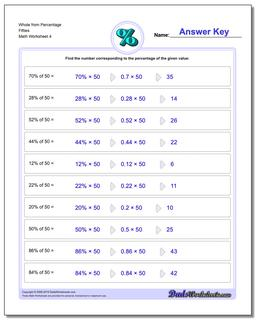# Math Worksheets: Percentages: Percentages: Whole from Percentage Fifties (Fourth Worksheet)## Whole from Percentage Fifties (Fourth Worksheet)

PropertyValue
DescriptionWhole from Percentage Fifties: Percent worksheets where students are given a whole number and a percentage, and must calculate the fraction of the whole represented. (Fourth Worksheet)
Resource TypeWorksheet